# Basic types, part 2

Contents
Video
• 11 minutes

Next we want to give you some more types to play with, so we can start to do things that are perhaps more interesting than arithmetic.

## Branching, generalized

First we should take another look at the `case` keyword. Let’s go back to that conditional statement that we wrote in the last section.

``````idk :: (Ord a, Num a) => a -> a
idk x = if (x < 10) then (negate x) else (x + 10)``````

We have already noticed that this branches on the result of an expression (`x < 10`) that evaluates to a `Bool` result. We also saw when we wrote the `spell` function how a function involving a `case ... of` can match columns of inputs to outputs.

The `if`-`then`-`else` form is great when what you have is a `Bool`, but it can’t take you any farther – it’s only useful for `Bool`. What comes after the `if` always has to be true or false. So we need to make sure we understand how to generalize this notion of branching and write expressions that can branch over anything.

What we’ll do now, just to get a little more `case` practice, is rewrite the `idk` function so that it uses a `case` expression instead of `if`-`then`-`else`. We’ll call it `idk2` just to give it a different name.

The type of the function will remain the same, so we can just copy the type declaration and change the name.

``idk2 :: (Ord a, Num a) => a -> a``

It’s the syntax of the function description itself that changes. Instead of saying `if (x < 10)`, we’ll say `case (x < 10) of`.

``````idk2 :: (Ord a, Num a) => a -> a
idk2 x =
case (x < 10) of
(...)``````

Instead of the `then` and `else` words, we’ll give a list of the possible Boolean result values and map them to the desired outputs, in a sort of tabular form like we did with `spell`.

``````idk2 :: (Ord a, Num a) => a -> a
idk2 x =
case (x < 10) of
True -> _____
False -> _____``````

Please take a moment to figure out what should go in each of the two blanks.

What we had previously written after the `then` keyword goes after the arrow for `True`, and the `else` corresponds to `False`. So it should end up looking like this:There are three expressions here we have parenthesized for clarity; in all three cases, the parentheses are optional. It never hurts to add them if you are unsure.

``````idk2 :: (Ord a, Num a) => a -> a
idk2 x =
case (x < 10) of
True -> (negate x)
False -> (x + 10)``````

This is called pattern matching. The patterns here are `True` and `False`.These probably don’t look like “patterns”, because they are extremely simple patterns. But once we see more complex examples, it may become more clear why what’s written on the left side of an `->` arrow is called a pattern. And the result is given by what’s on the right side of the arrow, based on which pattern matches the result of `x < 10` for a given `x`.

## Maybe

Let’s look next at another type that is very useful in Haskell and can be thought of as encoding a kind of two-valued logic. The type is called `Maybe` and, instead of choosing between false and true, it gives us a choice between nothing and something.

``data Maybe a = Nothing | Just a``

The biggest difference between `Bool` and `Maybe` is that `Maybe` has a type parameter.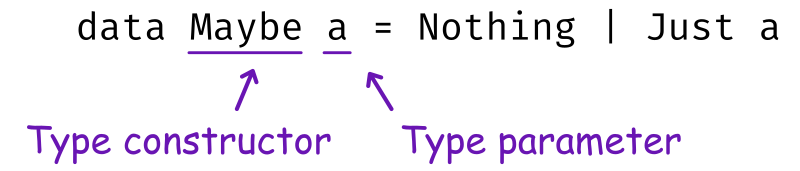The `Maybe` part of this, because it has a parameter, is described most precisely not as a type, but as a type constructor.

The `a` is the type parameter in this definition.Sometimes we use more descriptive names for type variables. But in this case, since this variable represents any type at all, and `Maybe` does not ascribe any particular meaning to it, there is really nothing to describe, no information that we might want to communicate via the choice of name. In cases like these, we conventionally use single letters `a`, `b`, `c` as parameter names. This is similar to the function parameters we’ve seen: it’s a variable that can stand for many different things. Whereas our `idk` function needed to be applied to a value of a numeric type, though, `Maybe` has to be applied to another type. Importantly, since there is no constraint on the `a` (that is, nothing like `Num a =>`) this `a` could be any type. `Maybe` can take any type and turn it into a new type `Maybe a` that has the possibility of being `Just` one value of that type or of being `Nothing`.

Just like what we saw in a function definition, a variable appears on both the left and right side of the `=`.

• The place where the variable appears to the left of the equals sign is called the binding; this assigns the name `a` to the variable.
• Wherever `a` appears on the right is where we are using what we bound on the left.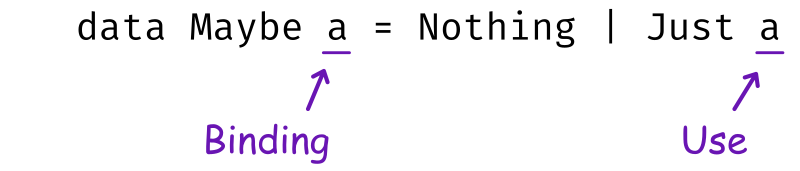The `a` in the `Just a` has to be a value of the type that we applied `Maybe` to.

Because `Just` has a parameter, we can use it like a function. For example, we can apply the `Just` constructor to the string `"Julie"`. Because we’ve given it a `String`, the type of the overall expression is `Maybe String`.

``Just "Julie" :: Maybe String``

For another example, suppose we apply the `Just` constructor to the value `3`. Just as we saw before, what we end up here is a polymorphic expression, because we haven’t specified exactly what type of number the `3` is. So the type of this expression is written as `Maybe a` – but it definitely does have to be a number, so there is also a `Num` constraint on the `a`.

``Just 3 :: Num a => Maybe a``

So why does this `Maybe` type exist – why would we want to take a perfectly good type and add a `Nothing` to it?

One thing that `Maybe` is useful for is giving us a way to “fail” functions by returning a result of `Nothing` which might mean we didn’t get an input that we consider valid. For instance, we might have wanted to use that in our `spell` function, to return `Nothing` instead of “I don’t know this number!” as the result for numbers that we don’t know how to write as words. We’ll be using it in this way a few lessons later when we write a small program.

## List

We’re also going to need lists for our forthcoming program. So, without dwelling on all the details, we are going to next take a brief look at the list datatype now.Because `[]` is a built-in type with special syntax in Haskell, this definition is not actually valid code that you could put in your `main.hs` source file. But this is what the definition would look like.

``data [] a = [] | a : [a]``

Relative to what we’ve seen so far, this looks pretty weird! For one thing the name of this type is `[]` not a pronounceable word like `Bool` or `Maybe`. However, it is a type constructor like `Maybe`.

We’ll write it this way with some extra spacing and add some annotations to make this easier to read: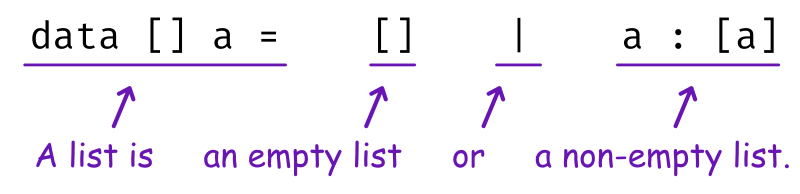When we write it down this way, we can see that it’s actually not so different from the definition of `Maybe`.

• The first thing, `[]`, is the name the thing being defined here, and `a` is the name of its parameter. For example, applied `[]` to `Integer` is the type list of integer, written as either `[] Integer` or, more commonly, `[Integer]`.
• There are exactly two possibilities for the form a list value can take, separately by the `|` that signifies or.
1. On one side, the `[]` constructor signifies an empty list, which is somewhat analogous to the `Nothing` constructor of `Maybe`.
2. On the right side of the pipe, the `(:)` constructor means “an `a` and a list of more `a`s.” That represents a list that has at least one item. That `(:)` operator is often pronounced as “cons”, and we might pronounce `a : [a]` as “`a` consed onto a list of `a`”.

Notice that these are what we call homogenous lists; if `a` has type `Integer`, then all of the items the list have type `Integer`. This is because in `a : [a]`, the `a` appears twice, representing the same type in each place.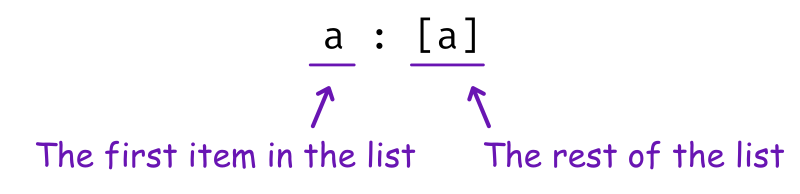The first item in the list has the same type `a` as the items in the rest of the list.

We’ll see more about how to use lists in later lessons. What we want you to keep in mind for now is the similarity between these types:

``````data Bool    =  False   | True
data Maybe a =  Nothing | Just a
data [] a    =  []      | a : [a]``````

These are all examples of sum types, meaning they have two possibilities – false or true, nothing or just, empty or cons. When working with any of those types, we’re going to be using `case` expressions to pattern match over the two possibilities.

This is the end of the first of four parts of the beginner crash course. That was a lot to take in, especially if you’re new to programming! You may want to take a break here. In the upcoming lessons, we’ll give an extended example to see how the ideas we’ve presented so far start to make sense in the context of an actual working program.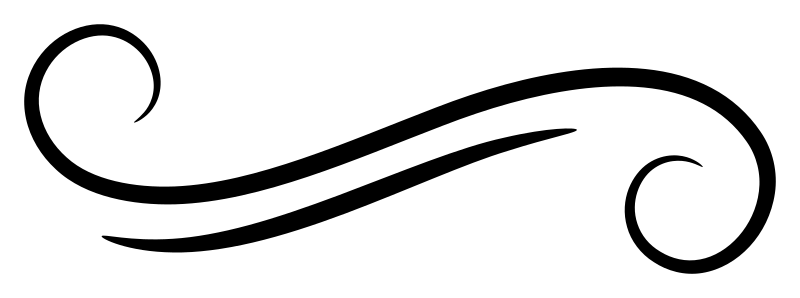Join Type Classes for courses and projects to get you started and make you an expert in FP with Haskell.